# My Latest paper published on Vixra – 3D Polytope Hulls of E8 4_21, 2_41, and 1_42

https://vixra.org/pdf/2005.0200v1.pdf

or also available directly from this website:

https://theoryofeverything.org/TOE/JGM/3D_Polytope_Hulls_of_E8-421-241-142.pdf

Using rows 2 through 4 of a unimodular 8x8 rotation matrix, the vertices of E8 421, 241, and 142 are projected to 3D and then gathered & tallied into groups by the norm of their projected locations. The resulting Platonic and Archimedean solid 3D structures are then used to study E8’s relationship to other research areas, such as sphere packings in Grassmannian spaces, using E8 Eisenstein Theta Series in recent proofs for optimal 8D and 24D sphere packings, nested lattices, and quantum basis critical parity proofs of the Bell-Kochen-Specker (BKS) theorem.

A few new Figures from the paper.

Now for a few new visualizations that are not in the paper…

# 3D visualization of E8 1_42 polytope

This is what I expect to be the first ever 3D visualization of the E8 1_42 polytope with 17280 vertices showing concentric hulls of Platonic solid related structures!E8 1_42 polytope with 17280 vertices showing concentric hulls of Platonic solid related structures! Vertex colors represent the overlap counts.Each of 74 concentric hulls based on 3D Norm’d vertex positions (with varying opacity in sets of 8). Vertex counts in each hull listed above.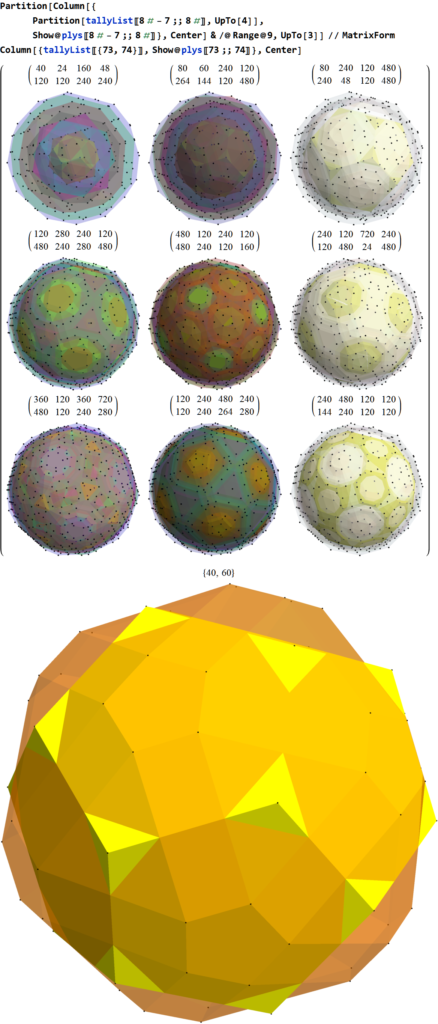9 sets of 8 concentric hulls plus the last 2 outer hulls, Vertex counts in each of 8 hull listed above. Notice this is a combination of two overlapped dodecahedrons (40) and a Nonuniform Rhombicosidodecahedron (60).

For the sake of completeness in visualization, see below for various projections to 2D. Click these links for a higher resolution PNG or the SVG version.

# Nested Lattices of E8 in Complex Projective 4-Space

I read an interesting article about a pattern discovered by Warren D. Smith (discussed at length here):

“The sum of the first three terms in the Eisenstein E_4(q)  Series Integers of the Theta series of the E8 lattice is a perfect fourth power: 1 + 240 + 2160 = 2401 = 7^4”

So I decided to visualize the 2401=1+240+2160 vertex patterns of E8 using my Mathematica codebased toolset based on some previous work I put on my Wikipedia talk page.

The image below represents various projections showing 6720 edges of the 240 E8 vertices, plus a black vertex at the origin, and the 2160 Witting Polytope E8 2 _ 41 vertices using the same projection basis (listed at the top of each image along with the color coded vertex overlaps). Click these links for a higher resolution PNG or the SVG version.

Some of the particular projections of the Witting Polytope may need 8D rotations applied to the basis vectors to find better symmetries with the Gosset, but this is a start using my standard set of projections.

The 240 vertices of the Gosset Polytope are generated using various permutations:

(* E8 4_21 vertices *)
e8421 = Union@Join[
Eperms8@{1, 1, 1, 1, 1, 1, 1, 1}/2,
perms8@{1, 1, 0, 0, 0, 0, 0, 0}];

The 2160 vertices of the Witting Polytope are generated using various permutations:

(* E8 2_41 vertices *)
e8241=Union@Join[
perms8[{1,0,0,0,0,0,0,0}4],
perms8[{1,1,1,1,0,0,0,0}2],
Eperms8[({2,0,0,0,0,0,0,0}+1)]]/4;

Another view shows just the 2160 Witting Polytope vertices. Click these links for a higher resolution PNG or the SVG version.

Another great source of visualizations on E8 and this Witting Polytope is here.

Now visualizing in 3D the structure in 3D using rows 2-4 of the E8->H4 folding matrix, we get:Witting Polytope with 2160 E8 2 _ 41 vertices sorted by Norm distance into 3D Platonic solid related concentric hull structures using rows 2-4 of the E8->H4 folding matrix. The last image shows overlap counts using colored vertices.

# Visualizing the concentric hulls of E8 with the 24-cells of H4 & H4Φ

I’ve had a number of related posts on this topic, but I wanted to present a few new pictures and PDF documents that combine the 7 concentric hulls forming Platonic solid related sets of E8 vertices projected to 3D with vertices of five pairs of 24-cell objects.

These break down the E8 structure into familiar 3D objects, such as the icosahedron with its dual the dodecahedron, the icosidodecahdron, and the 16-cell with its dual the 8-cell (aka. Tesseract) combined to create the self-dual 24-cell.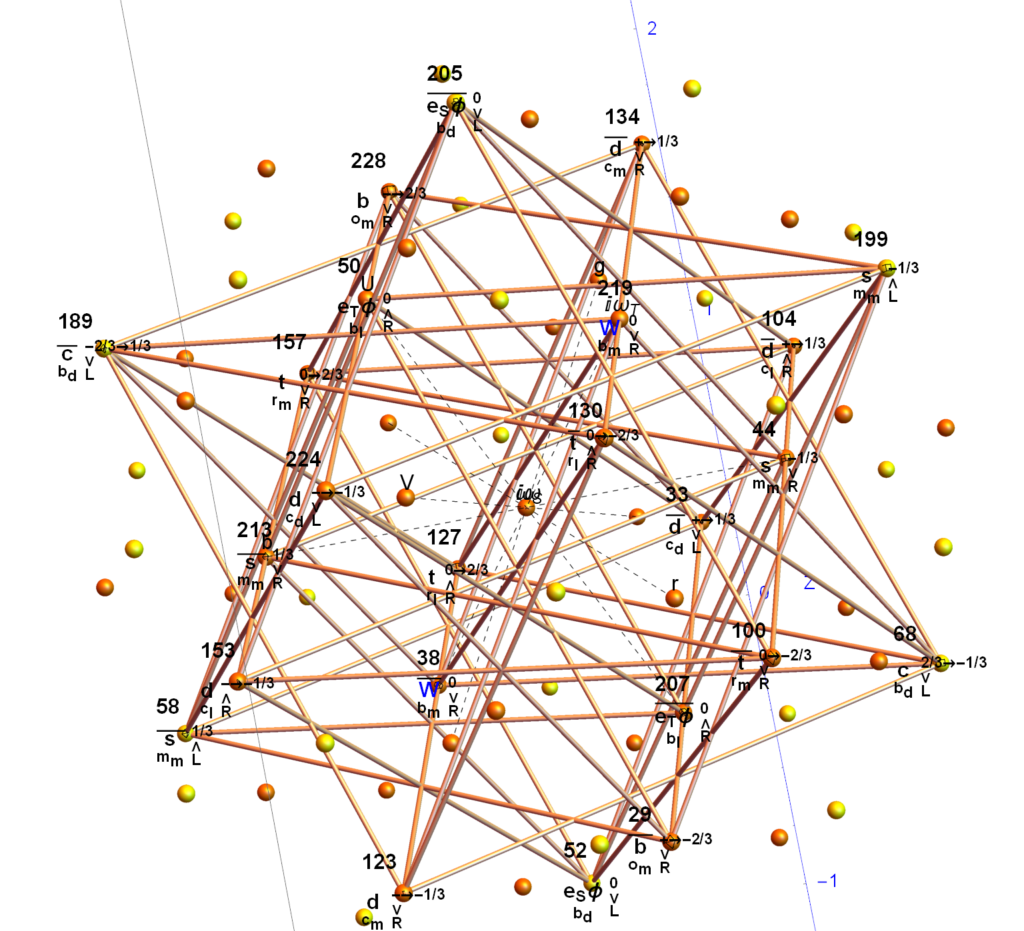The yellow spheres are those of the outer hull of singular 30 vertices of an icosidodecahedron. The orange spheres are pairs of icosahedron or dodecahedron vertices. The edges with numbered vertices form one of five 24-cells in the 120 vertex H4Φ scaled 600-cell within E8. Specifically, it is the 3rd rotation (of 4 π/5 rotations) within the 96 vertices of the snub-24-cell. You can see that 6 of the yellow vertices connect to the 24-cell. Rotating that 24-cell four times in 3-space by π/5 gives the connections to the rest of the vertices in H4Φ and completes the 30 vertex icosidodecahedron . The same is true for the 120 vertices of H4 using the corresponding 24-cell in H4.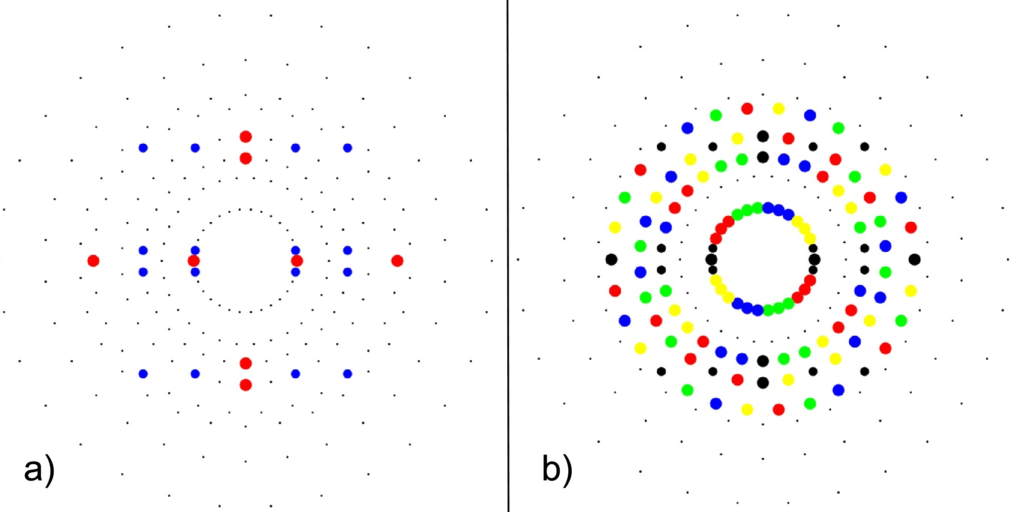a) 24-cell highlighting the 16-cell (red on-axis vertices) and 8-cell (blue off-axis vertices)b) Snub 24-cell highlighting four π/5 rotations of the 24-cell (black) in red, green, blue, yellow.

The following paper hulls-24cells-combined.pdf (13MB) & interactive Mathematica Notebook hulls-24cells-combined.nb (34MB) contains a comprehensive set of images that show the contents of the 7 concentric hulls of Platonic solid related shapes as well as their integration with one of the rotations of 24-cells in H4 and H4Φ in E8 (the same one used in the image above).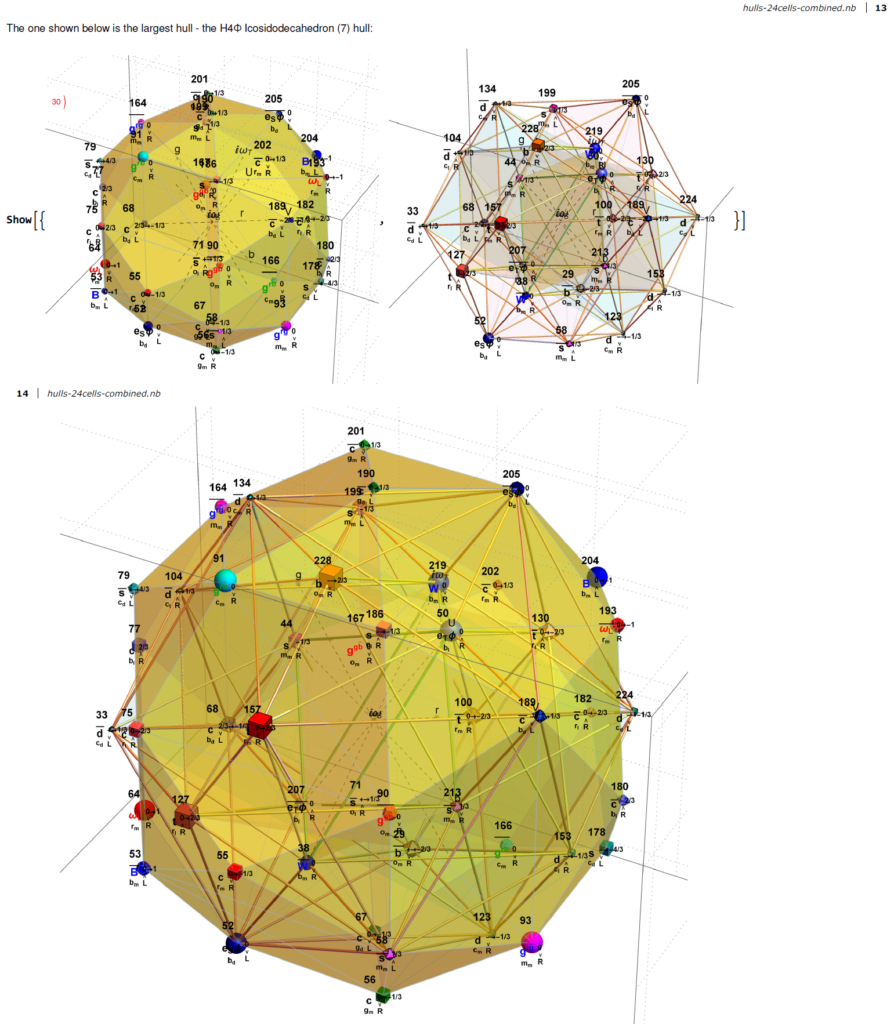Snapshot from the paper showing the 3rd rotation of the snub 24-cell and the icosidodecahedron of H4Φ

In the E8 Petrie projection, every hull (each with 2 or 4 overlapping vertices) pair into left/right patterns. See the set of four icosahedrons that occupy the 3rd hull in both 2D and 3D (the yellow edge sets belongs to the H4Φ and the blue sets belong to H4) :

The pair of dodecahedrons that occupy the 5th hull in both 2D and 3D (the yellow edge set belongs to the H4Φ and the blue belong to H4) :

Below is an animation that cycles through the sequence of 2D Petrie Projections of the pairs of hulls. One cycle shows each frame individually and the other builds the E8 Petrie from the previous 2D hull.

Below are images of the left (H4) and right (H4Φ) 2D Petrie projections of the hulls (which are defined by the Norm’s of 3D projected vertices using the E8->H4 folding matrix rows 2-4 as basis vectors). The two 30 vertex 4 & 7 Icosidodecahedron hulls and four 0th hull (points) are omitted leaving the gaps in the diagram. When these gap vertices are combined with the 48 3rd hull Icosahedrons (above), they make up the 112 integer D8 group assigned to the Bosons (48) and 2nd generation Fermions (64) in the physics model described below.

The combined set of hulls projected using the rows of the folding matrix as basis vectors is shown below from previous work:

This paper hull-list-3b.pdf (4MB) & interactive Mathematica Notebook hull-list-3b.nb (20MB) contains the visualizations and detail E8 vertices associated with each Platonic solid related concentric hulls. This paper 24cell-list-3b.pdf (6MB) & interactive Mathematica Notebook 24cell-list-3b.nb (20MB) contains the visualizations and detail for the five 24-cells in each H4 & H4Φ. Both of these papers also have detail information about its assigned physics particle based on a modified A.G. Lisi model (shown below). This paper describes these particle assignment symmetries in more detail.

# Latest Paper – Unimodular rotation of E8 to H4 600-cells

## Please see my latest paper that describes some advances in understanding the E8 to H4 rotation matrix

https://theoryofeverything.org/TOE/JGM/Unimodular-Rotation-of-E8-to-H4.pdf

Abstract: We introduce a unimodular Determinant=1 8×8 rotation matrix to produce four 4 dimensional copies of H4 600-cells from the 240 vertices of the Split Real Even E8 Lie group. Unimodularity in the rotation matrix provides for the preservation of the 8 dimensional volume after rotation, which is useful in the application of the matrix in various fields, from theoretical particle physics to 3D visualization algorithm optimization.

# Working with E8+++

I wanted to confirm some work being done with E11 (or more specifically the Extended E8+++), so I used my “VisibLie” notebook (which includes the “SuperLie” package for analyzing Lie Algebras) to get the following information:

I first created the Dynkin diagram which produces the Cartan Matrix: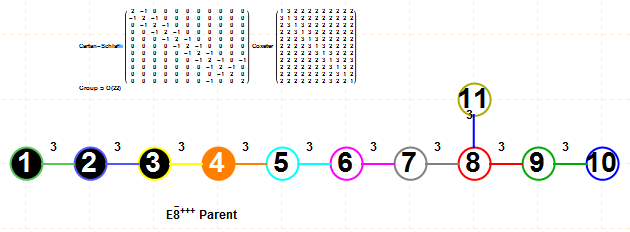This allows the evaluation of the EigenSystem of the Cartan matrix as follows: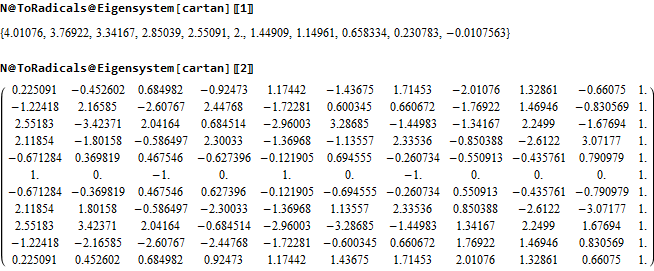As well as using the SuperLie package to evaluate the Positive Roots, Weights and Heights of the E8+++ (The first 80 are shown. For the full list in .pdf click here or click on the image below):The 4870 positive roots up to height 47 generate a Hasse diagram as follows (with 3 sections zoomed in):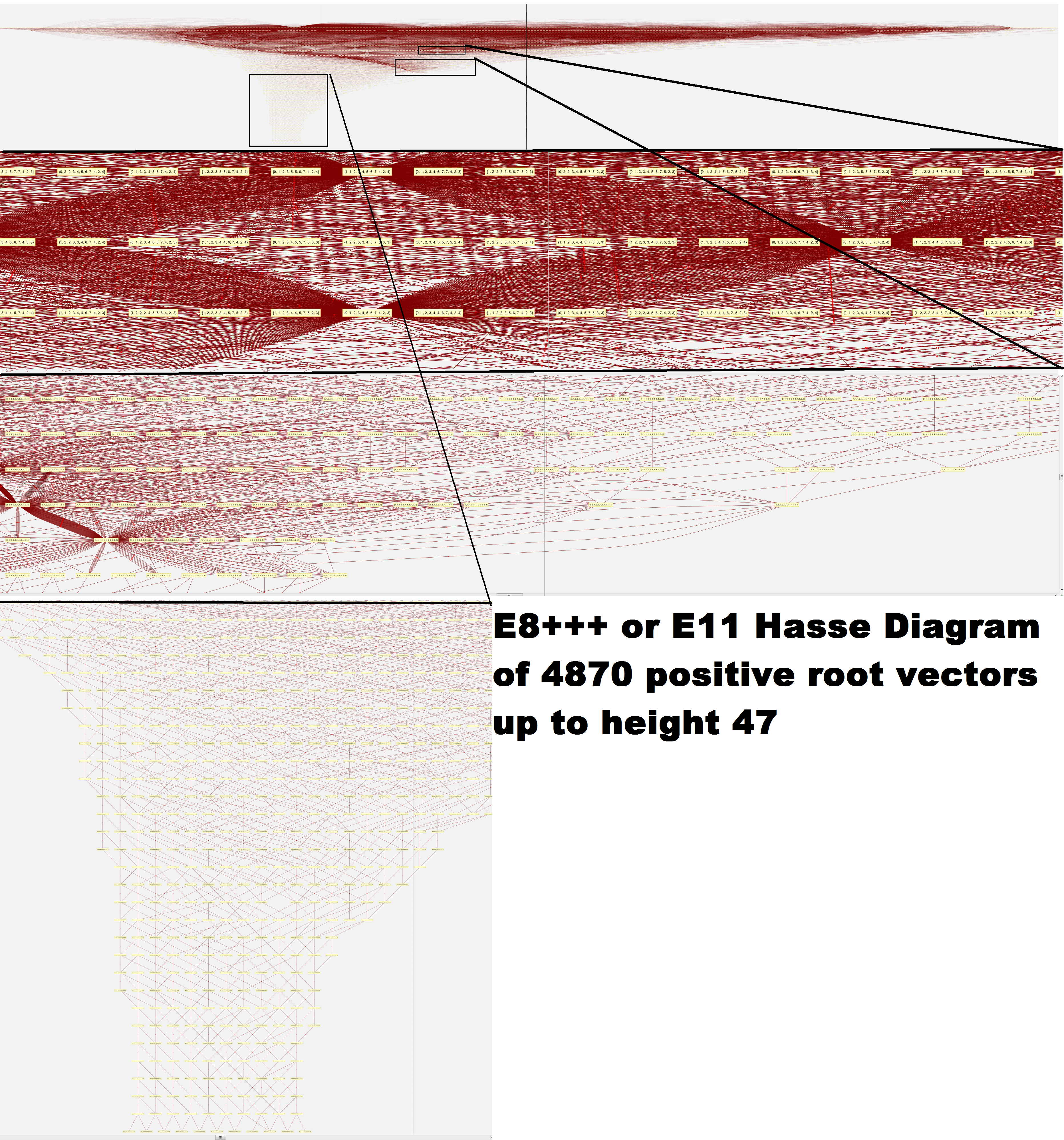I am working on replication of the Petrie projection by A.G. Lisi based on his prescription using his basis vectors for 2D projection and a Simple Roots Matrix: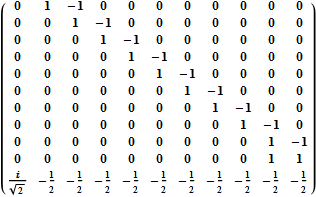Adjusting this E11 Simple Roots Matrix (srmE11) to match the Dynkin diagram above and then verifying it creates the same Cartan matrix, we have: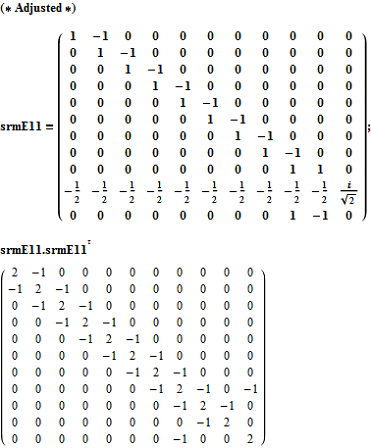To get the 9740 E8+++ vertices, we simply use the dot product of the transpose of srmE11 against the positive and negative root vectors (first 50 shown):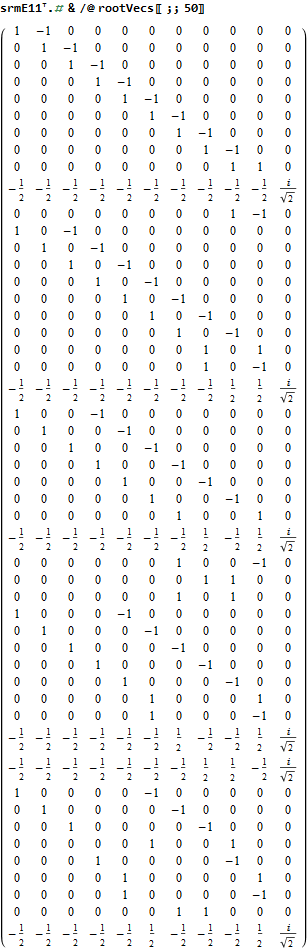A first cut at plotting these involves projecting them to a 2D using the dot product of the 2 basis vectors provided, which gives the following ListPlot: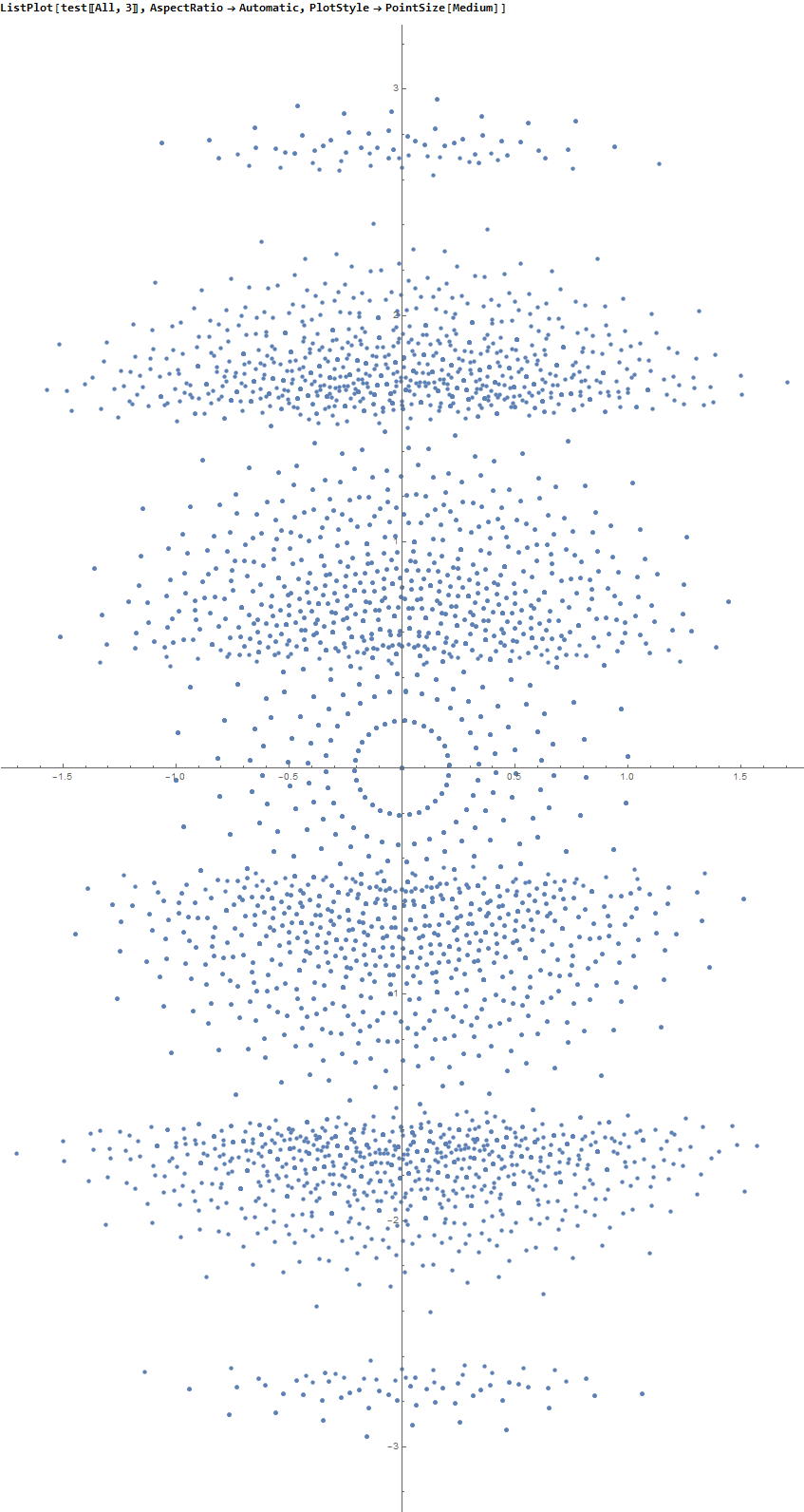The limited results from SuperLie are consistent with the image given by Lisi on the Bee’s BackReaction blog, after eliminating the vertical “levels” in my raw plot.

Email me if you want more detail, see errors, or would like to help.

Greg
JGMoxness@theoryofeverything.org

# Mapping the fourfold H4 600-cells emerging from E8: A mathematical and visual study

My latest paper is now available here or on Vixra.

{abstract}
It is widely known that the E8 polytope can be folded into two Golden Ratio (Φ) scaled copies of the 4 dimensional (4D) 120 vertex 720 edge H4 600-cell. While folding an 8D object into a 4D one is done by applying the dot product of each vertex to a 4×8 folding matrix, we use an 8×8 rotation matrix to produce four 4D copies of H4 600-cells, with the original two left side scaled 4D copies related to the two right side 4D copies in a very specific way. This paper will describe and visualize in detail the specific symmetry relationships which emerge from that rotation of E8 and the emergent fourfold copies of H4. It will also introduce a projection basis using the Icosahedron found within the 8×8 rotation matrix. It will complete the detail for constructing E8 from the 3D Platonic solids, Icosians, and the 4D H4 600-cell. Eight pairs of Φ scaled concentric Platonic solids are identified directly using the sorted and grouped 3D projected vertex norms present within E8. Finally, we will show the relationship of the Beordijk-Coxeter Tetrahelix emerging from the Petrie projection’s concentric rings of 30 vertices of the H4 600-cells.

This paper builds off of some recent work here, and here.

Below are a few figures from that paper.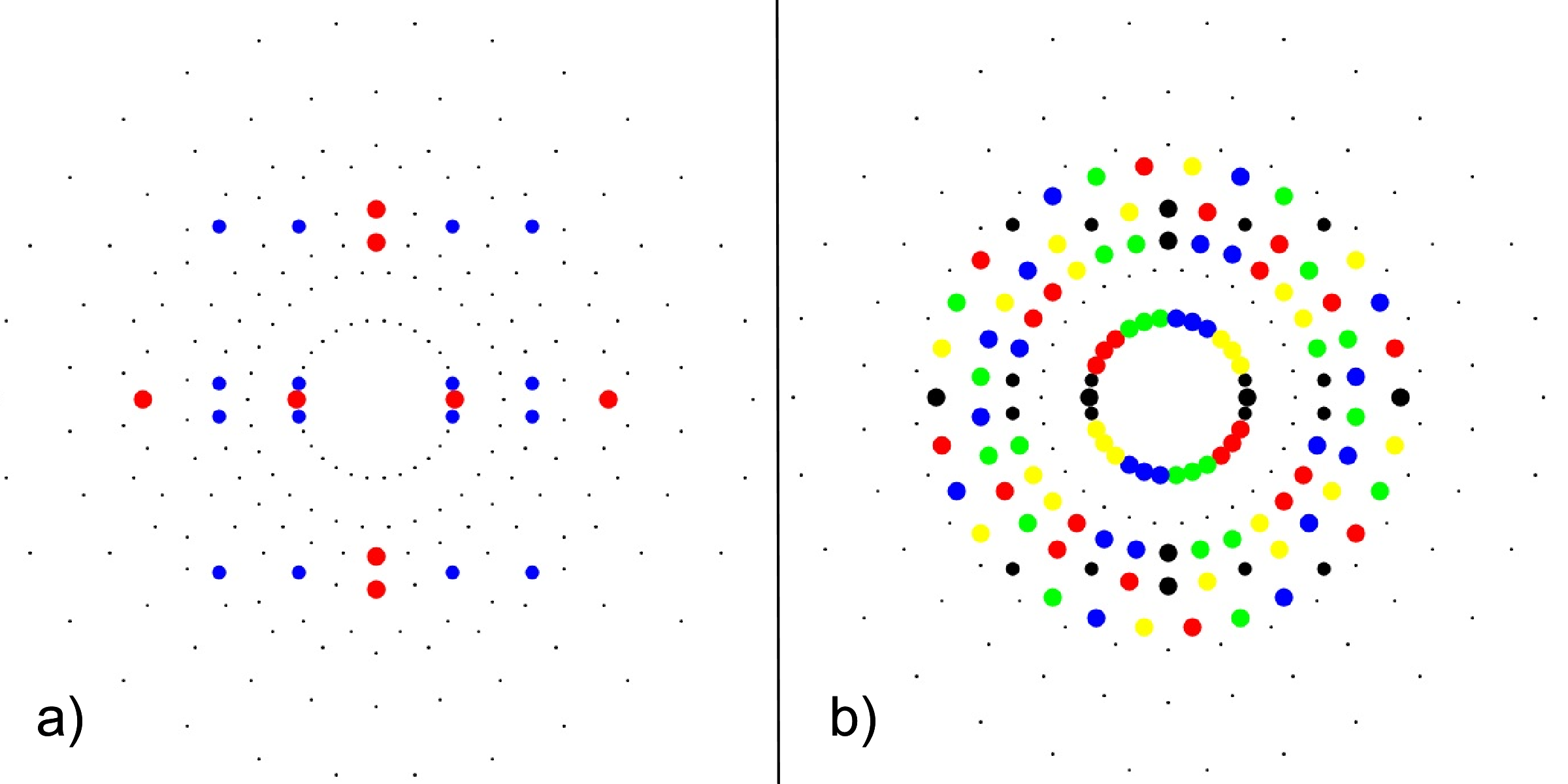FIG. 3: a) 24-cell highlighting the 16-cell (red on-axis vertices) and 8-cell (blue off-axis vertices), b) Snub 24-cell highlighting four pi/5 rotations of the 24-cell (black) in red, green, blue, yellow.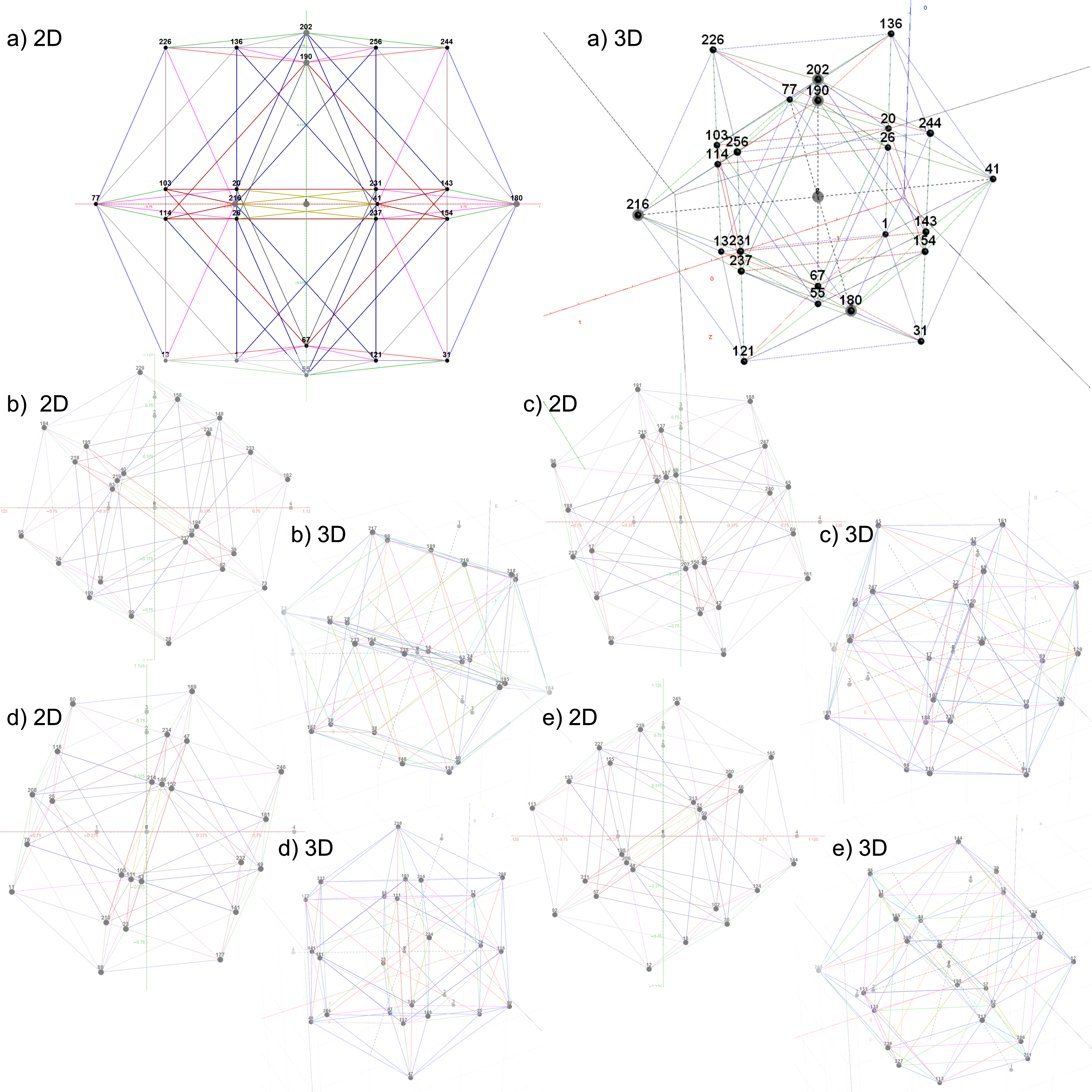FIG. 4: The 5 24-cell decomposition of one 600-cell, each with 2D and 3D vertex numbered projections including edges; a)
24-cell, b-e) Snub 24-cells from four pi/5 rotations of the 24-cell in a)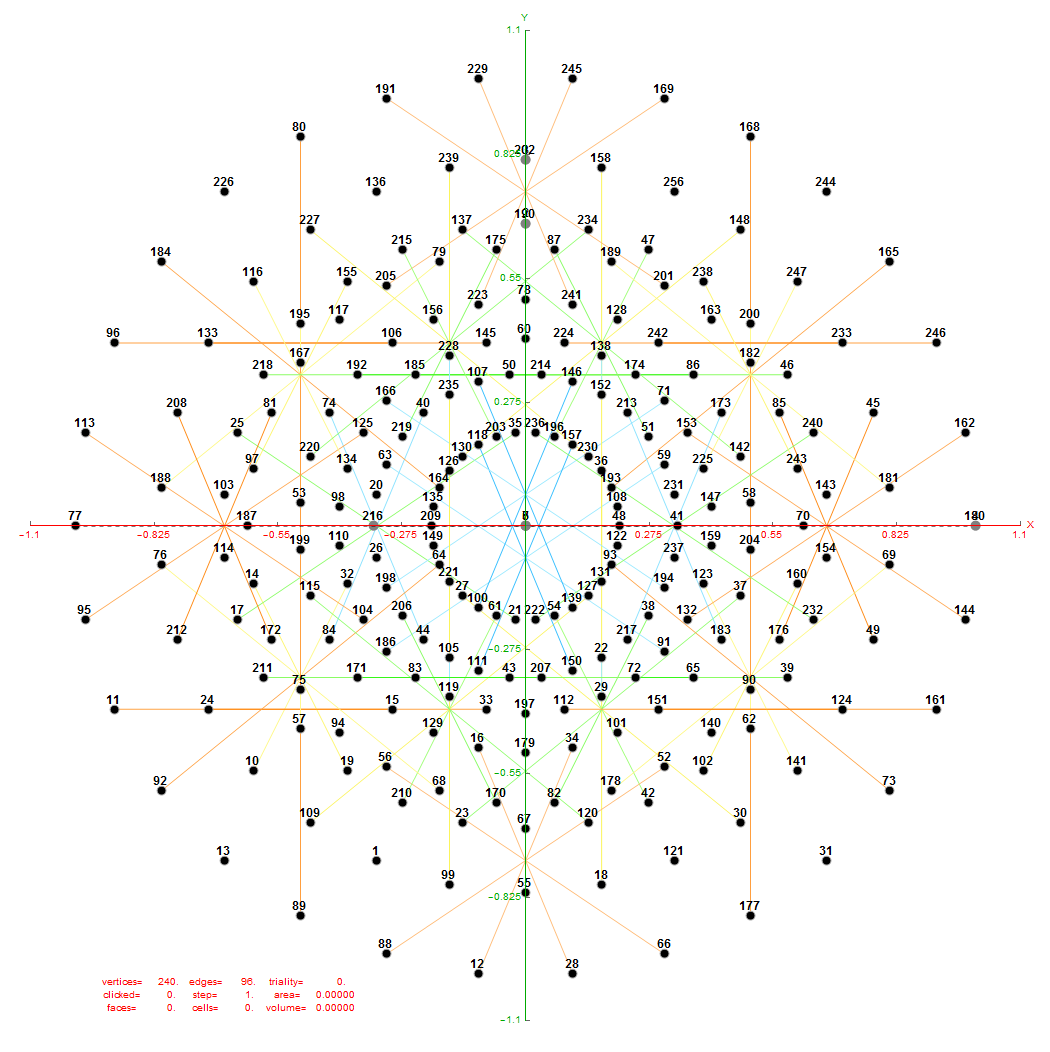FIG. 6: Vertex numbered Petrie projection of the rotated E8, showing the 96 edges of 8D norm l = 2Φ which links the Snub 24-cell H4 and H4Φ (L<->R) vertices (Note: the 8 excluded positive E8 8-Orthoplex (or equivalently, the H4 and H4Φ 4-Orthoplex) “generator vertices” are shown as larger gray labeled axis dots which overlap their darker black E8 vertices)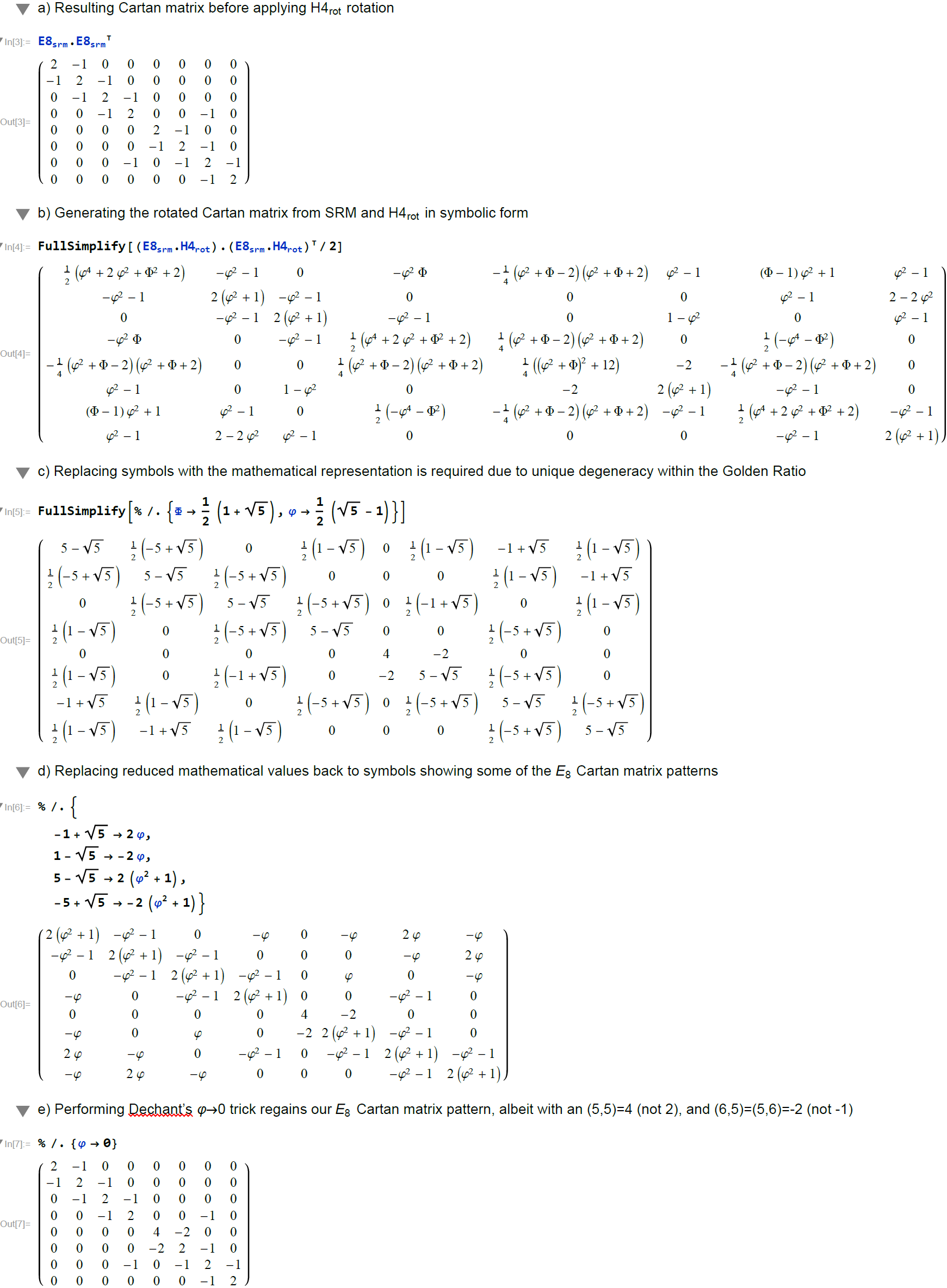FIG. 7: Symbolic analysis using MathematicaTM comparing the Cartan matrix before and after rotating the simple roots matrix (E8srm) used to create it (Note: the resulting Cartan matrix is not precisely that of E8, even after applying the Dechant’s Φ=0 trick)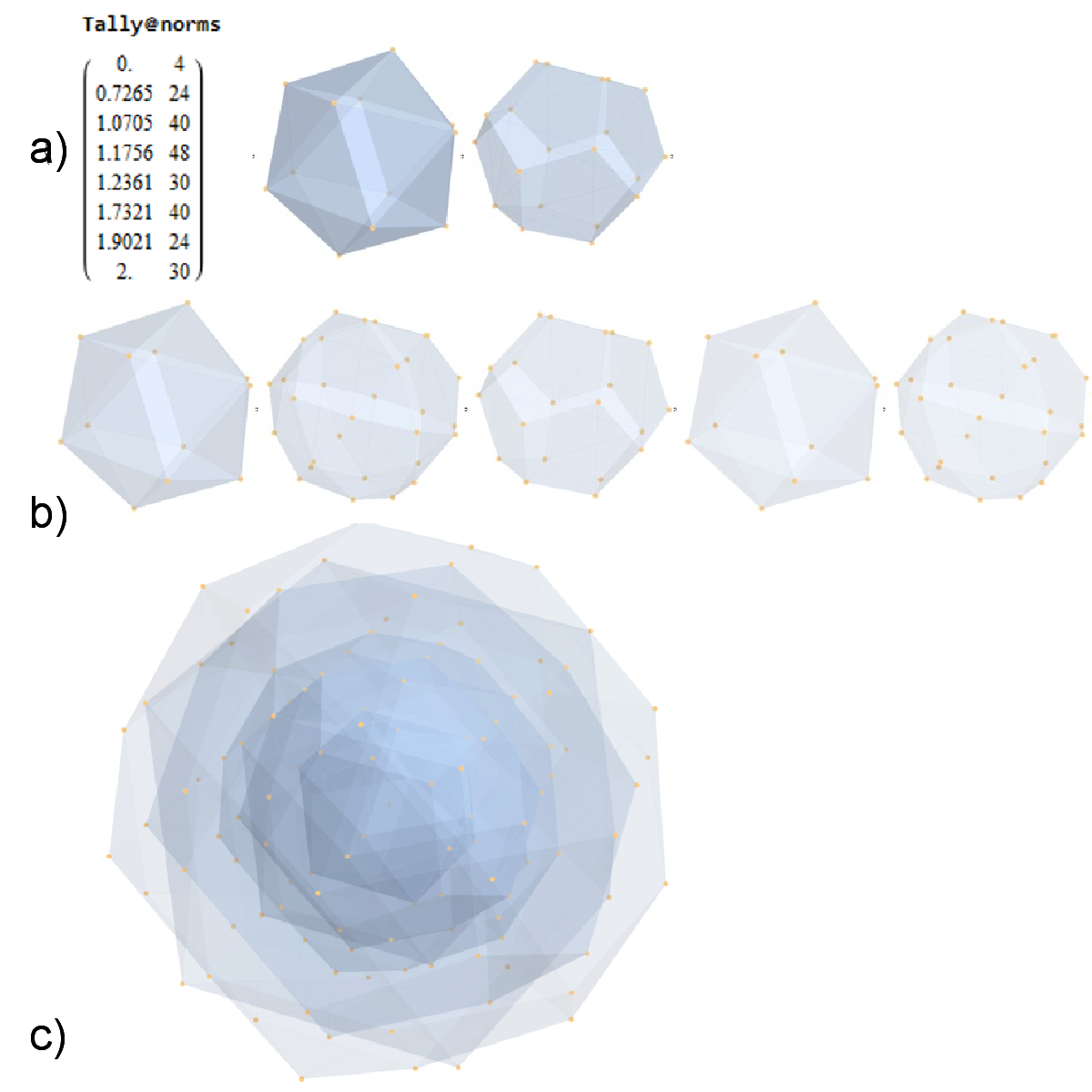FIG. 8: a) Sorted list of the vertex norms with their grouped vertex counts. b) 3D surface models for each of the 7 hulls of vertices c) Combined 3D surface model with increasing transparency for each successive hull.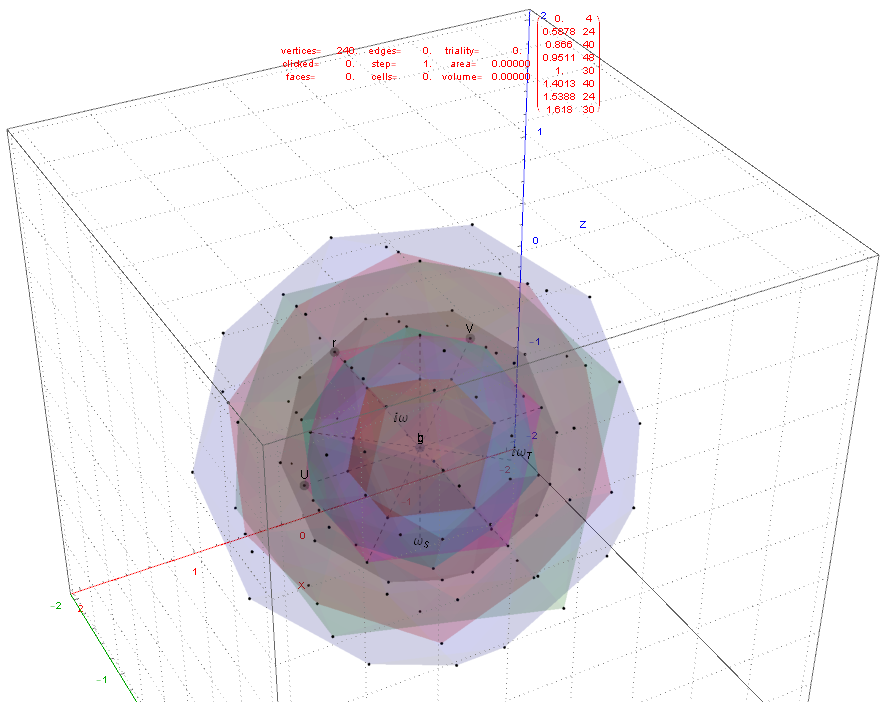FIG. 11: 3D projection using an orthonormal basis on H4 and H4Φ vertices (i.e. the rotated E8)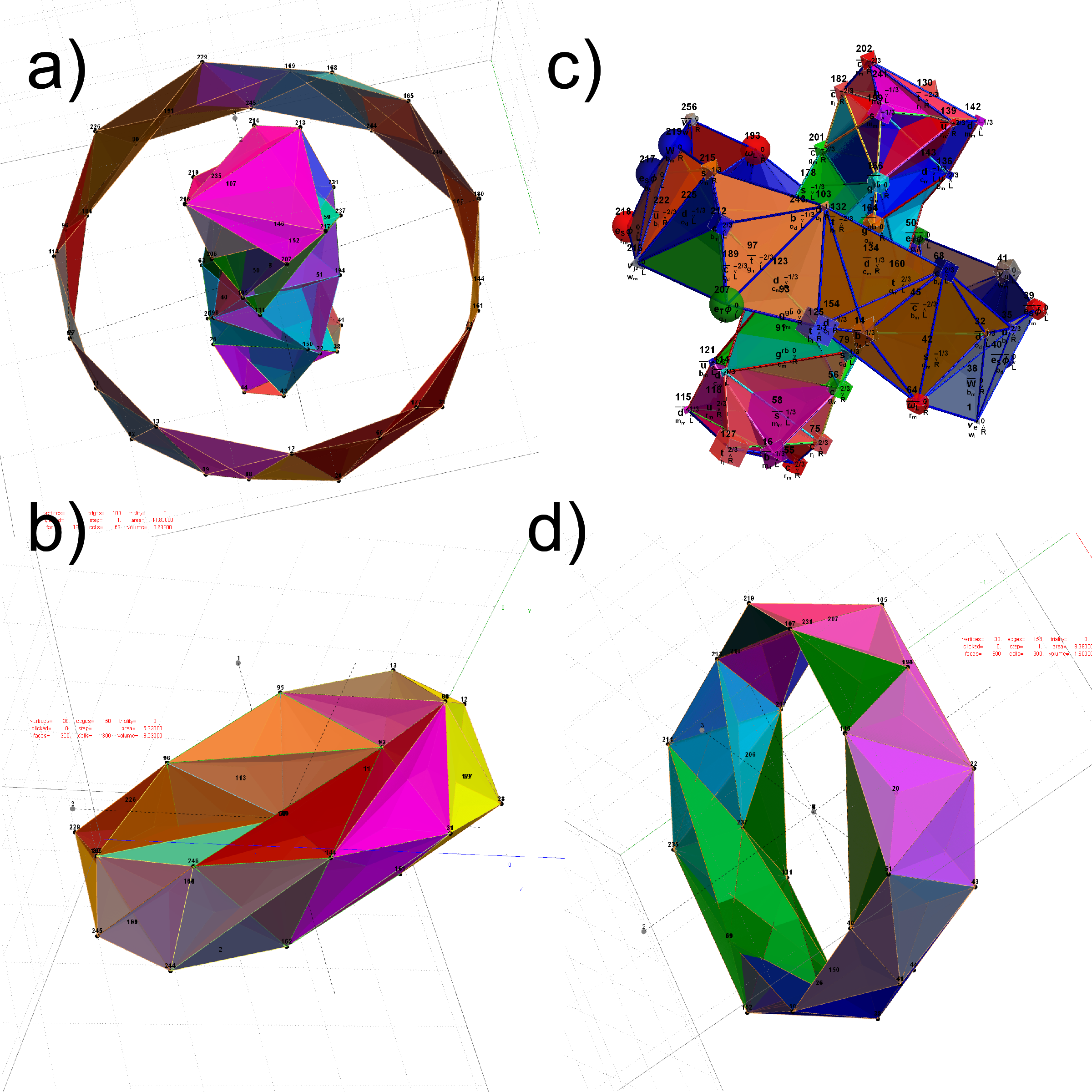FIG. 12: The Beordijk-Coxeter Tetrahelix emerging from the 8 concentric rings of 30 E8 and H4+H4Φ Petrie projection vertices; a) H4 inner and outer ring in 3D, b) with vertex size, shape, color assigned from physics model, c-d) inner and outer ring in orthonormal projection of H4Φ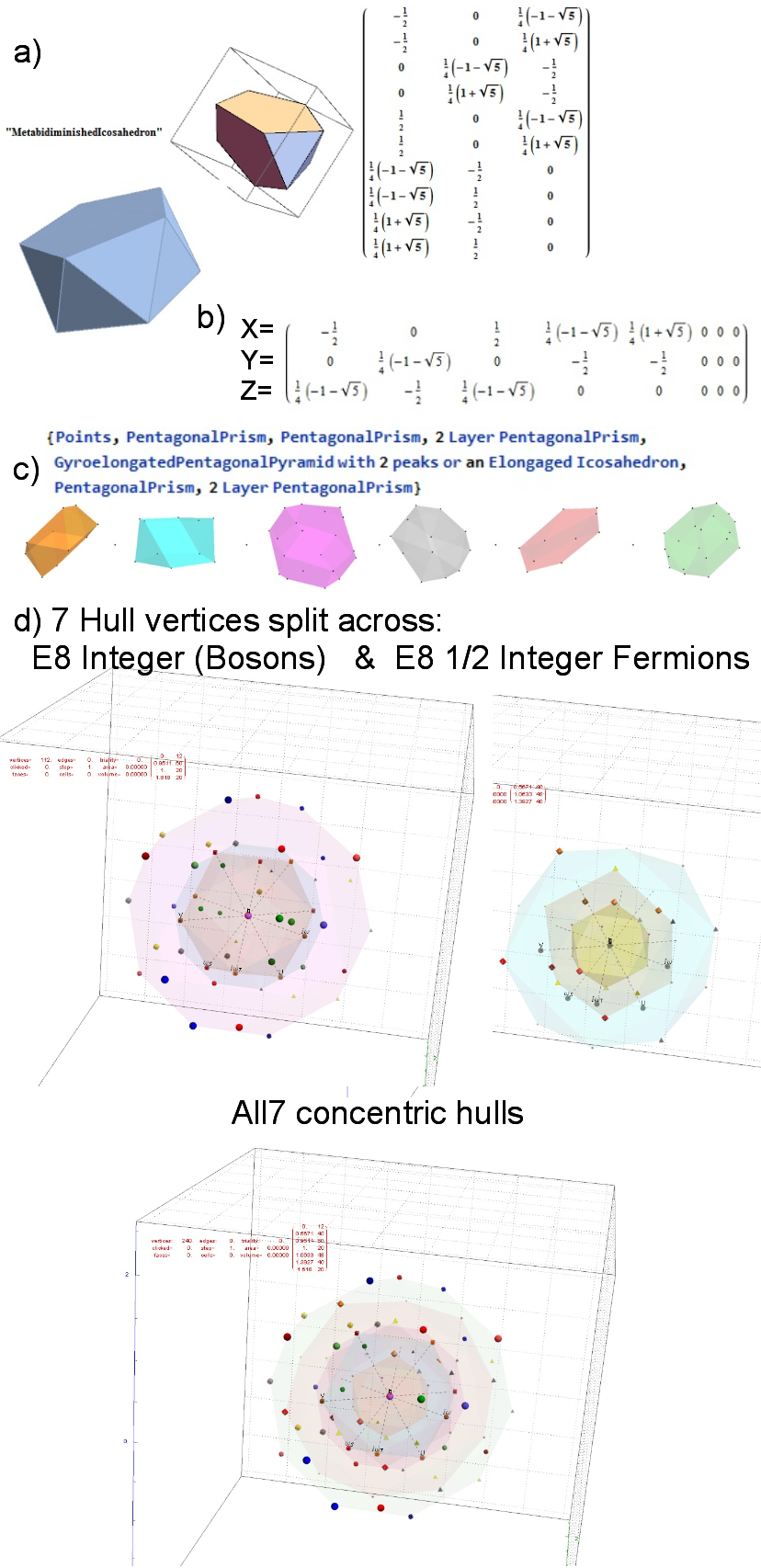FIG. 15: MetabidiminishedIcosahedron (with its negated vertices gives the GyroelongatedPentagonalPyramid) Crystal Projection Prism projecting E8 a) the crystal prism geometry and vertices, b) the selected basis vectors, c) the individual 3D projected concentric hull objects, d) the combined set of concentric objects with progressively increasing transparency and tally of vertex norms.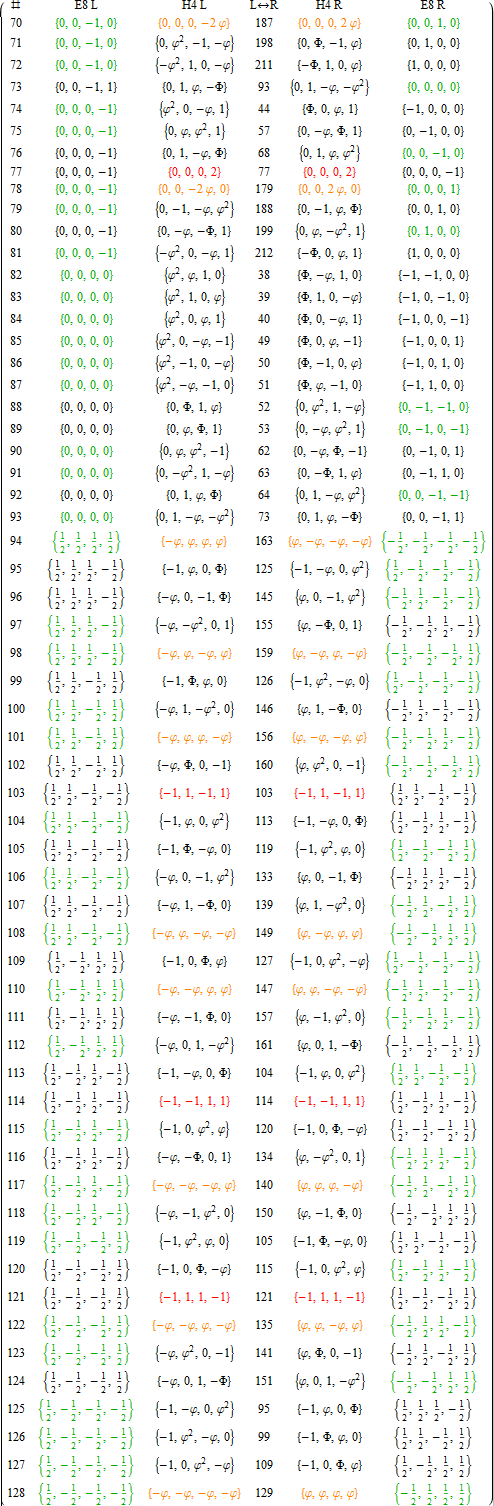APPENDIX A: LIST OF E8 AND ITS ROTATED H4 AND H4Φ VERTICES
This table splits the E8 and its rotated H4 vertices into L/R 4D elements.

1. Table structure
The first column is an SRE E8 vertex index number derived from sorting the E8 vertices by their position based on the 256=2^8 binary pattern from the 9th row of the Pascal triangle (1, 8, 28, 56, 70, 56, 28, 8, 1) and its associated Cl8 Clifford Algebra. This construction is described in more detail in . The odd groups (1,3,5,7,9) with (1,26,70,26,1) elements (respectively) are the 128 1/2 integer vertices. The even groups (2,4,6,8) with (8,56,56,8) elements (respectively) are the 112 integer vertices along with the 16 excluded 8 generator (and 8 anti-generator) vertices (2-9 and 248-255) with permutations of (1; 0; 0; 0; 0; 0; 0; 0) (a.k.a. the 8-Orthoplex), such that they indicate the basis vectors used for projecting the polytope.

Only the first half of the 240 vertices is shown, since the last half is simply the reverse order negation of the vertices
in the first half (e.g. the E8 vertex n=10 has as its’ negation 257-n=247). The middle column labeled L<->R indicates the vertex reference number that contains the same L as the R (and equivalently, the same R as the L, interestingly enough).

2. Table color coding
The E8 L/R columns’ green color-coded elements indicate that the 4D vertex rotates into the smaller H4 600-cell.
Conversely, the E8 L/R columns’ black color-coded elements indicate the 4D vertex rotates into the larger H4Φ
600-cell.

The H4 L/R columns’ red color-coded rows are 24-cell elements that always self-reference and are always members of the scaled up 600-cell H4Φ. The H4 L/R columns’ orange color-coded rows are 24-cell elements that always reference the negated elements in the range of 129-256 and are always members of the smaller 600-cell H4.

# E8 (2_41, 4_21, and 1_42) projected to various Coxeter planes

This is a shot of the relevant Mathematica code snippets that produce the images:E8 4_21 (with projection basis, edges, vertex overlap colors & counts) (click here for very large SVG):E8 2_41 (with projection basis, vertex overlap colors & counts) (click here for very large SVG):

# 3D Printed E7 1_32 Polytope Projected to the E6/F4 Coxeter Plane

The 3D model of the polytope: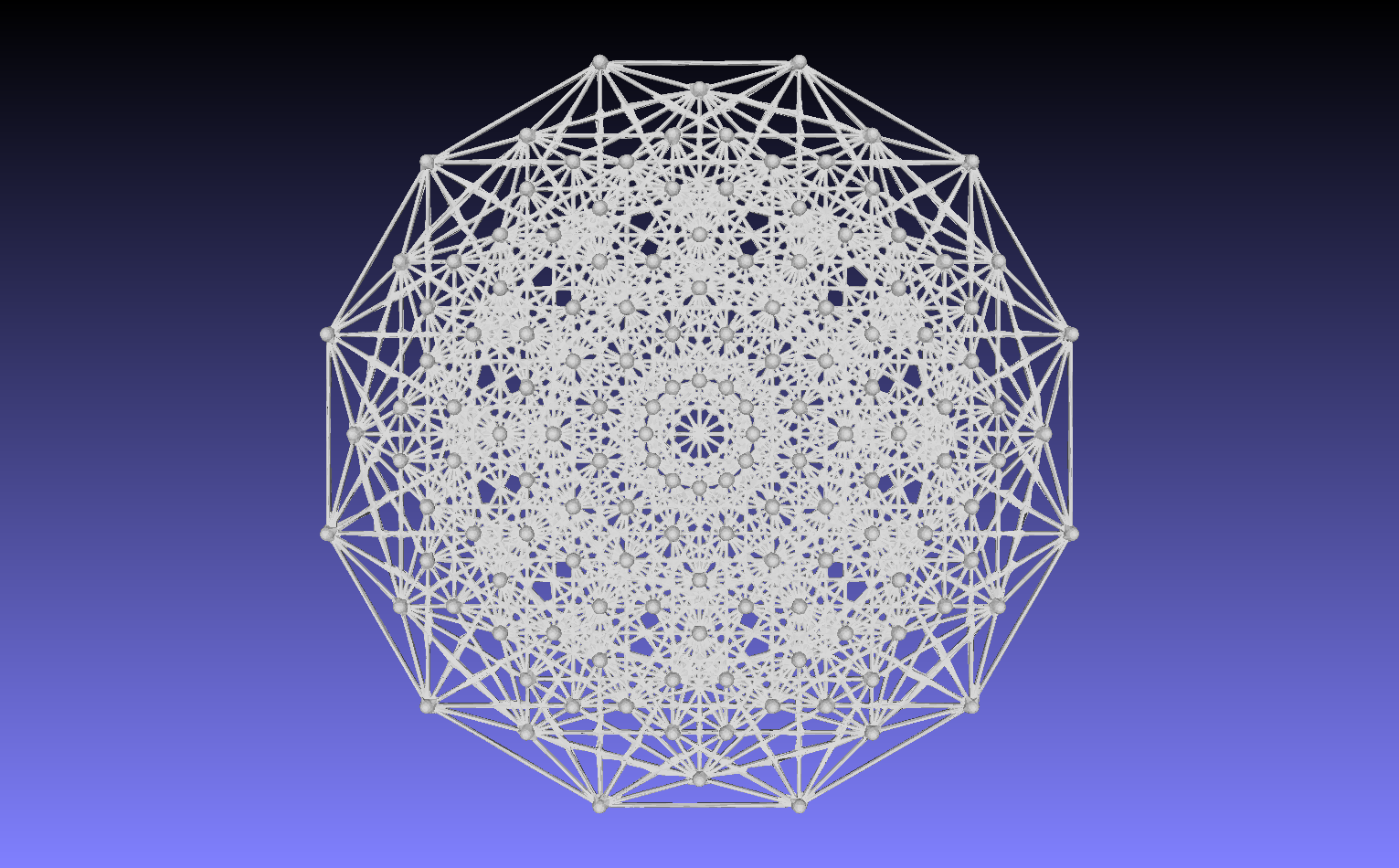3D printed in raw bronze:
Front: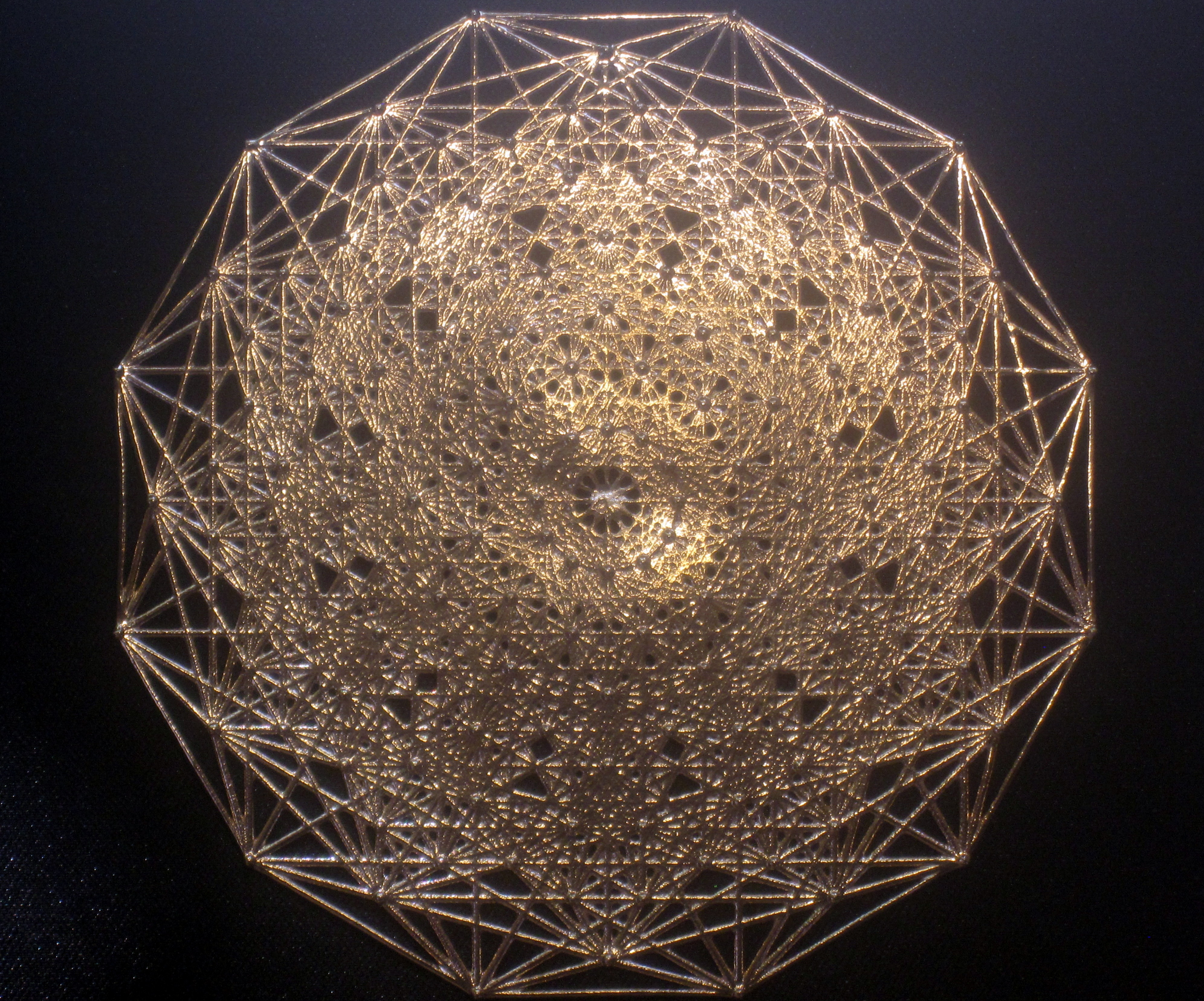Back: